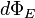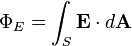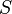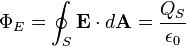# Electric flux facts for kids

Kids Encyclopedia Facts

Imagine an electric field E passing through a surface. Consider an infinitesimal area (dA) on that surface across which E remains constant. Also assume that the angle between E and dA is i. The electric flux is defined as EdAcos(i). E and dA are vectors. Flux is the dot product of E and dA. Using full vector notation, the electric flux$d\Phi_E\,$ through a small area$d\mathbf{A}$ is given by$d\Phi_E = \mathbf{E} \cdot d\mathbf{A}$

The electric flux over a surface S is therefore given by the surface integral:$\Phi_E = \int_S \mathbf{E} \cdot d\mathbf{A}$

where E is the electric field and dA is a differential area on the surface$S$ with an outward facing surface normal defining its direction.

For a closed Gaussian surface, electric flux is given by:$\Phi_E = \oint_S \mathbf{E} \cdot d\mathbf{A} = \frac{Q_S}{\epsilon_0}$

where QS is the net charge enclosed by the surface (including both free and bound charge), and ε0 is the electric constant. This relation is known as Gauss' law for electric field in its integral form and it is one of the four Maxwell's equations.

Electric flux is not affected by charges that are not within the closed surface. But the net electric field, E, in the Gauss' Law equation, can be affected by charges that lie outside the closed surface. Gauss' Law is true in all situations, but people can only use it to calculate when high degrees of symmetry exist in the electric field. Examples include spherical and cylindrical symmetry. Otherwise, the calculations are too hard to do by hand and must be worked out using a computer.

Electrical flux has SI units of volt metres (V m), or, equivalently, newton metres squared per coulomb (N m2 C−1). So, the SI base units of electric flux are kg•m3•s−3•A−1.

## Other pagesElectric flux Facts for Kids. Kiddle Encyclopedia.